# Tutorial: NHANES T2D and Ovarian Datasets

## Clinical Trial Generalizabilility Assessment Methods – Comparing populations in clinical studies and Ovarian Datasets

We are using the 2003-2012 Type 2 diabetes National Health and Nutrition Examination Survey data as an example to demonstrate the methods involved in the clinical trial generalizability assessment. The total sample size of Type 2 diabetes patients is N = 2695 and we defined it as the General Population.

First, we applied three clinical trial inclusion criteria, based on the “Efficacy Evaluation of Different Medication Combination in Type 2 Diabetes Treatment Study”” (ClinicalTrials.gov Identifier: NCT01076842), to the dataset and categorized the patients as two groups: trial participants and non-participants.

• Age is from 18 to 90 years old (variable name: RIDAGEYR)
• BMI is less than 40 kg/m2 (variable name: BMXBMI)
• Hemoglobin A1C is between 7.5% and 10% (variable name: LBXGH)

We will use the three groups: general population (GenPop), trial participants (Trial), and non-participants (NonPart) in the further analysis.

``````T2D = read.csv("/Users/gjxjohn/Desktop/FSU STAT - TANG/Dr. He-Research/Dropbox/Generalizability Tutorials/Xiang/NHANES_T2D_tutorial_10242019.csv", header = TRUE)
``````##    SEQN RIAGENDR RIDRETH1 LBXGH BMXBMI RIDAGEYR
## 1 21043        1        4   5.4  62.77       31
## 2 21093        2        2  12.1  28.97       64
## 3 21099        1        3   5.8  40.41       75
## 4 21104        1        4   6.5  29.33       70
## 5 21112        1        5   7.3  21.65       76
## 6 21118        1        1   5.5  25.76       66``````

## 2. Data Cleaning

To exam if there is missing values in the dataset, we can use “sum(is.na)” to calculate the number NAs in the dataset, then use “na.omit” to delete any rows that contain NA values to use a complete case for analysis.

``sum(is.na(T2D))``
``##  0``
``# There is no missing values in this dataset.``

## 3. Create trial participants and non-participants subgroups

Create the trial participants and non-participants groups based on the three inclusion criteria mentioned above.

``````# install.packages('dplyr')
library(dplyr)``````
``````##
## Attaching package: 'dplyr'``````
``````## The following objects are masked from 'package:stats':
##
##     filter, lag``````
``````## The following objects are masked from 'package:base':
##
##     intersect, setdiff, setequal, union``````
``````T2D = mutate(T2D, GROUP = ifelse(T2D\$BMXBMI<40 & T2D\$LBXGH>7.5 & T2D\$LBXGH<10 & T2D\$RIDAGEYR>18 & T2D\$RIDAGEYR<90, "Trial", "NonPart"))
table(T2D\$GROUP)``````
``````##
## NonPart   Trial
##    2150     545``````
``````# 545 type 2 diabetes patients met the inclusion criteria and are grouped as Trial Participants.
# The rest 2150 patients are grouped as Non-participants.``````

## 4. Normality Check

Before deciding on which clinical trial generalizabilility assessment method that we should preform, it is important to check the normality of a variable’s distribution. In R, the histogram plot will give an indication of the shape of the distribution. A density curve smooths out the histogram and can be added to the graph.

``````hist(T2D\$BMXBMI,probability=T)
lines(density(T2D\$BMXBMI),col=2)``````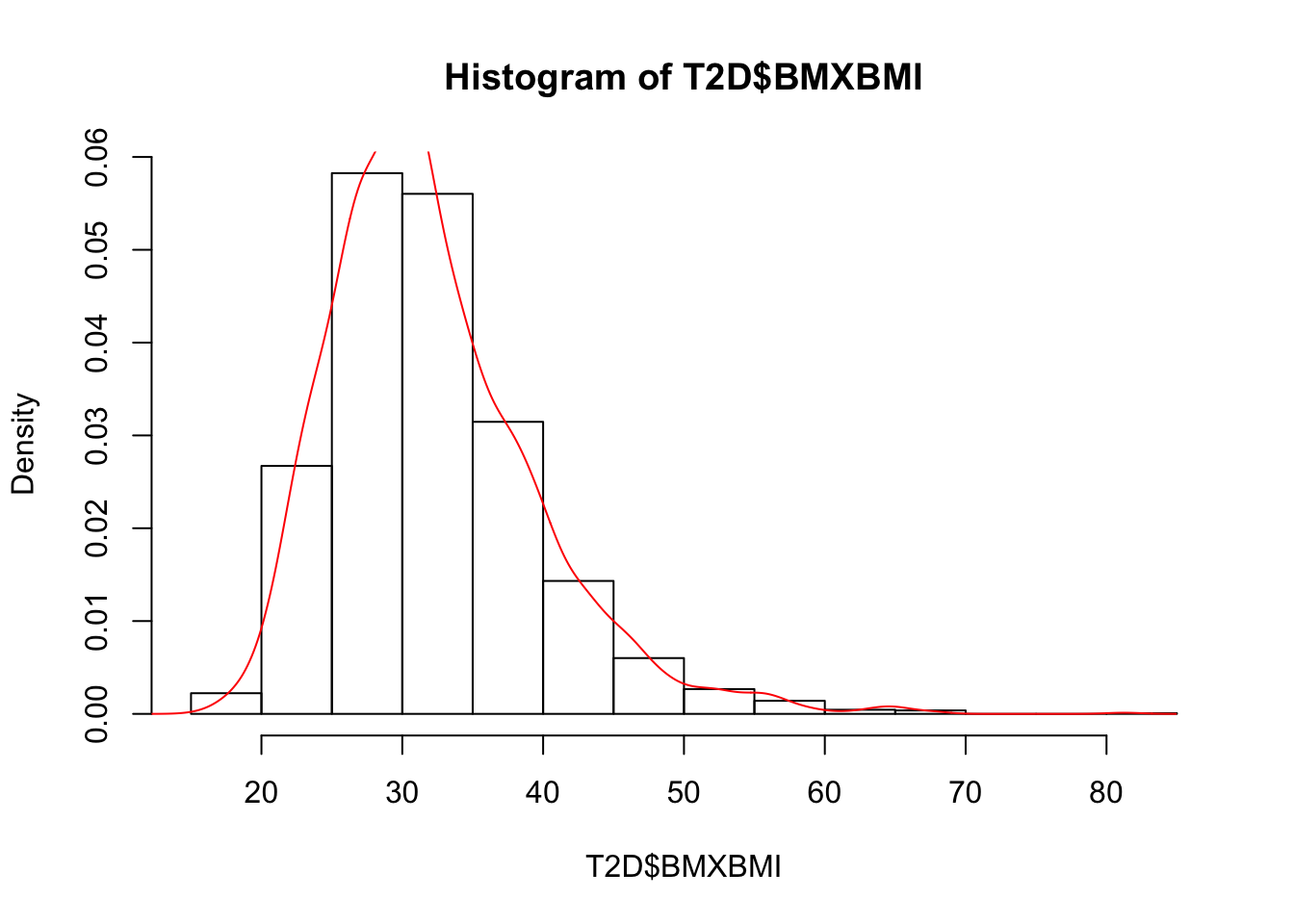``# The histogram plot shows the Body Mass Index data is skewed right, which is not normally distributed.``

Additionally, the normal Q-Q plot is an alternative graphical method of assessing normality to the histogram and is easier to use when there are small sample sizes.

``````qqnorm(T2D\$BMXBMI)
qqline(T2D\$BMXBMI,col=2)``````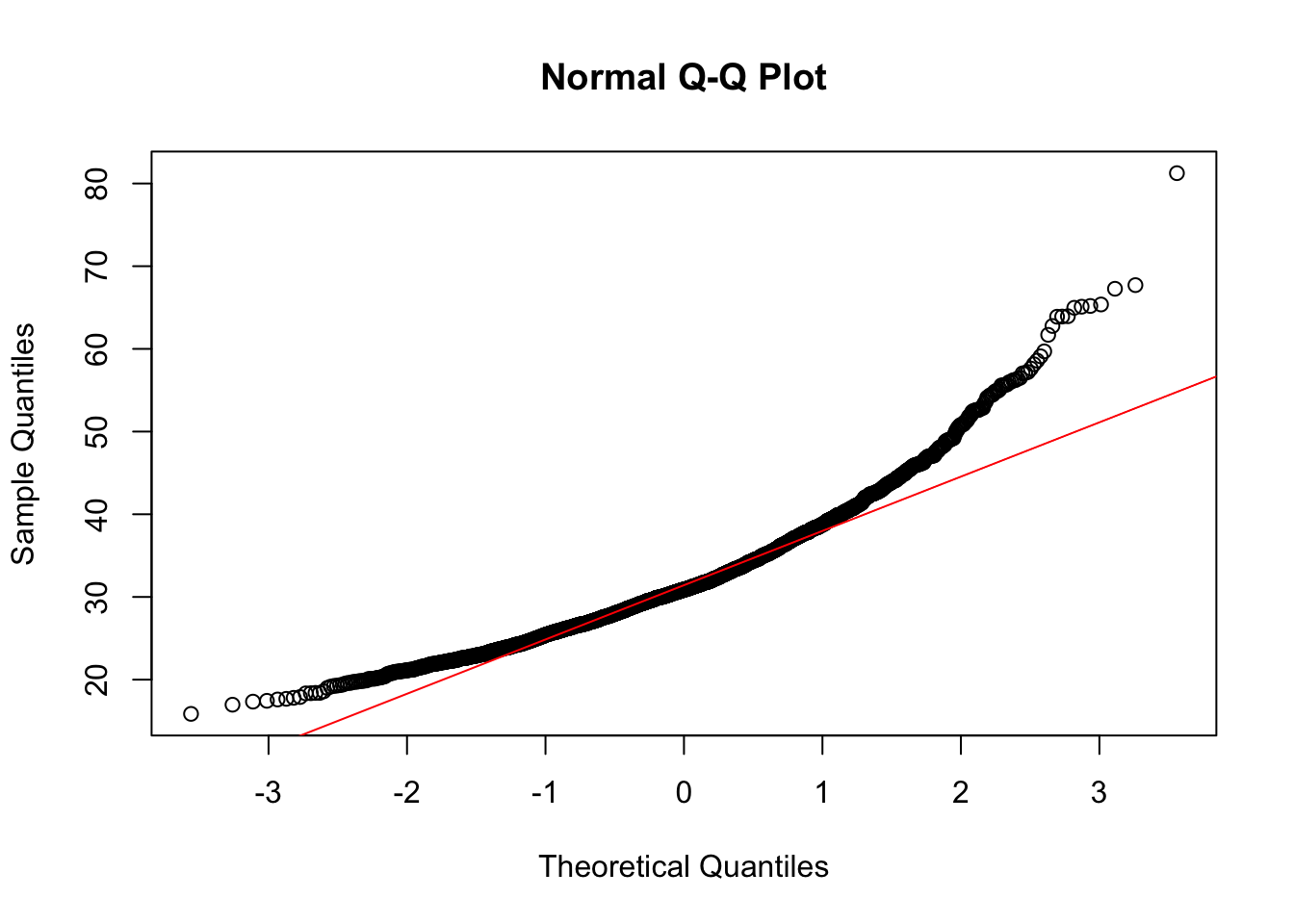``# The Q-Q plot shows the Body Mass Index data is not normally distributed. ``

However, sometimes it is hard to tell if the data is normally distributed or not. We can conduct a statistical test to get a more confirmative conclusion of the normality, Shapiro-Wilk Normality Test can be used (if sample size is between 3 and 5000). If p-value is greater than 0.05, it indicates the data is normally distributed at the 95% significant level. Otherwise, the data is skewed.

Anderson-Darling normality test can be used with more than 5000 sample size.

``shapiro.test(T2D\$BMXBMI)``
``````##
##  Shapiro-Wilk normality test
##
## data:  T2D\$BMXBMI
## W = 0.93931, p-value < 2.2e-16``````
``````# p-value is much less than 0.05.
# The null hypothesis is rejected, meaning the data is not normally distributed.

# install.packages('nortest')
library(nortest)

``````##
##  Anderson-Darling normality test
##
## data:  T2D\$BMXBMI
## A = 32.676, p-value < 2.2e-16``````
``# Anderson-Darling normality test also shows p-value less than 0.05.``

## 5. Compared Baseline Characteristics

Baseline information includes demographics (gender, age, ethnicity, etc.) and clinical characteristics (Body Mass Index, Hemoglobin A1C, etc.)

Categorical Variables Comparisons

• Chi-square Test

Chi-Square Test, also written as χ2 test, is used to test the associations of two categorical variables having only two subcategories, which means the output table should be 2-by-2.

For example, we want to know if gender (male and female) affect the clinical trial enrollment. In other words, if male or female patients are more likely to be enrolled in the type 2 diabetes trial.

``````T2D\$RIAGENDR <- factor(T2D\$RIAGENDR, levels = c(1,2), labels = c("Male", "Female"))
# Change the coded 1, 2 to labels 1=Male, 2=Female

trial_gender = table(T2D\$RIAGENDR, T2D\$GROUP) # Create a 2x2 table
trial_gender``````
``````##
##          NonPart Trial
##   Male      1058   304
##   Female    1092   241``````
``chisq.test(trial_gender)``
``````##
##  Pearson's Chi-squared test with Yates' continuity correction
##
## data:  trial_gender
## X-squared = 7.2485, df = 1, p-value = 0.007096``````
``````# p-value is less than 0.05, meaning there is a significant difference between male and female patients in regard to the clinical trial enrollment.
# Male are more likely to be enrolled in the type 2 diabetes trial``````
• Fisher’s Exact Test

Fisher’s Exact Test is used in the analysis of contingency tables. The test is used when the two categorical variables have more than two subcategories or relatively small sample sizes. Although in practice it is employed when sample sizes are small, it is valid for all sample sizes.

For example, we want to know if race/ethnicity affect the clinical trial enrollment. In other words, if a particular ethnic group is more likely to be enrolled in the type 2 diabetes trial.

``````T2D\$RIDRETH1 <- factor(T2D\$RIDRETH1, levels = c(1,2,3,4,5), labels = c("Mexican American", "Other Hispanic", "Non-Hispanic White", "Non-Hispanic Black", "Other Race"))
# Change the coded to labels

trial_race = table(T2D\$RIDRETH1, T2D\$GROUP) # Create a table for fisher's exact test
trial_race``````
``````##
##                      NonPart Trial
##   Mexican American       399   138
##   Other Hispanic         177    49
##   Non-Hispanic White     835   175
##   Non-Hispanic Black     599   138
##   Other Race             140    45``````
``fisher.test(trial_race, simulate.p.value=TRUE)``
``````##
##  Fisher's Exact Test for Count Data with simulated p-value (based on
##  2000 replicates)
##
## data:  trial_race
## p-value = 0.001499
## alternative hypothesis: two.sided``````
``````# p-value is less than 0.05, meaning there is a significant association between ethinicity and the clinical trial enrollment.
# Non-Hispanic White and Black are less likely to be enrolled in the clinical trial compared to Hispanics and other race.``````

Numerical Variables Comparisons

A. Normally Distributed Data

Since the T2D dataset does not have a variable that is normally distributed, we randomly generated a new variable “SleepTime” for each patient that follows a normal distribution with mean = 6.8 hours per day and standard deviation = 1.6.

``````n = nrow(T2D) # Get the number of rows
SLEEP = rnorm(n, mean = 6.8, sd = 1.6) # Randomly generate a normally distributed variable
T2D\$SLEEP = SLEEP # Add the new variabe into the T2D dataset
head(T2D) # the modified T2D dataset - appending GROUP and SLEEP variables``````
``````##    SEQN RIAGENDR           RIDRETH1 LBXGH BMXBMI RIDAGEYR   GROUP     SLEEP
## 1 21043     Male Non-Hispanic Black   5.4  62.77       31 NonPart  9.184180
## 2 21093   Female     Other Hispanic  12.1  28.97       64 NonPart 11.532708
## 3 21099     Male Non-Hispanic White   5.8  40.41       75 NonPart  6.288587
## 4 21104     Male Non-Hispanic Black   6.5  29.33       70 NonPart  4.319138
## 5 21112     Male         Other Race   7.3  21.65       76 NonPart  5.796460
## 6 21118     Male   Mexican American   5.5  25.76       66 NonPart  6.367090``````
• Student’s t-test

A t-test is most commonly applied when the test statistic would follow a normal distribution. It can be used, for example, to determine if the means of two sets of data are significantly different from each other.

For example, we want to know if there is a difference between the clinical trial participants or not in regard to sleep time at 95% confidence level.

``t.test(SLEEP ~ GROUP, data = T2D, conf.level = 0.95)``
``````##
##  Welch Two Sample t-test
##
## data:  SLEEP by GROUP
## t = 1.1402, df = 831.2, p-value = 0.2545
## alternative hypothesis: true difference in means is not equal to 0
## 95 percent confidence interval:
##  -0.06539875  0.24668522
## sample estimates:
## mean in group NonPart   mean in group Trial
##              6.818138              6.727495``````
``# The p-value is greater than 0.05. It means sleep time does not affect the type 2 diabetes clinical trial enrollment.``
• Z-test

Z-test is based on the standard normal distribution. For each significance level, the Z-test has a single critical value (for example, 1.96 for 5% two tailed) which makes it more convenient than the Student’s t-test which has separate critical values for each sample size. Therefore, many statistical tests can be conveniently performed as approximate Z-tests if the sample size is large or the population variance is known. If the population variance is unknown (and therefore has to be estimated from the sample itself) and the sample size is not large (n < 30), the Student’s t-test may be more appropriate.

For example, we have known that the mean of sleep time is 6.8 and the variance is 2.6 for the general population (the total sample N=2695). We want to compare the average sleep time of the clinical trial participant with the general population.

``````# Define the z.test function with standardized z-score
# x = sample dataset
# popmu = population mean
# popvar = population variance

z.test = function(x,popmu,popvar){
one.tail.p = NULL
z.score = round((mean(x) - popmu) / sqrt(popvar / length(x)), 3) # round to three decimal places
one.tail.p = round(pnorm(abs(z.score), lower.tail = FALSE), 3)
# Set up the output display
cat("z = ", z.score, "\n",
"one-tailed probability =", one.tail.p, "\n",
"two-tailed probability = ", 2*one.tail.p) # cat = concatenate and print
}

# Get the mean of sleep time in the general population
mean(T2D\$SLEEP)``````
``##  6.799808``
``````# Get the variance of sleep time in the general population
var(T2D\$SLEEP)``````
``##  2.696253``
``````# Subset the sleep time of the trial participants
trial_sleep = T2D[which(T2D\$GROUP=='Trial'), 8]

# Conduct the one-sample Z-test
z.test(trial_sleep, mean(T2D\$SLEEP), var(T2D\$SLEEP))``````
``````## z =  -1.028
##  one-tailed probability = 0.152
##  two-tailed probability =  0.304``````
``# p-value is greater than 0.05, which means the average sleep time of the clinical trial participants is not different from the mean of the general population.``
• F-test

Student’s t-test is used to compare the means of two groups, while F-test is used to assess whether the variances of two groups (A and B) are equal. The R function var.test() can be used to compare.

For example, we want to know if the variances of the clinical trial participants and non-participants, in regard to sleep time, are the same at 95% confidence level.

``var.test(SLEEP ~ GROUP, data = T2D)``
``````##
##  F test to compare two variances
##
## data:  SLEEP by GROUP
## F = 0.96847, num df = 2149, denom df = 544, p-value = 0.6263
## alternative hypothesis: true ratio of variances is not equal to 1
## 95 percent confidence interval:
##  0.8454734 1.1034369
## sample estimates:
## ratio of variances
##          0.9684696``````
``# The p-value is greater than 0.05. It means the variances of sleep time between the clinical trial participants and the non-participants have no difference.``
• Analysis of Variance (ANOVA)

Analysis of variance (ANOVA) is a collection of statistical models and their associated estimation procedures (such as the “variation” among and between groups) used to analyze the differences among group means in a sample. ANOVA provides a statistical test of whether two or more population means are equal.

There are two functions we can use to conduct an ANOVA test, namely oneway.test() and aov().

For example, we want to know if there are any differences in regard to slepping time among different ethnicity groups.

``oneway.test(SLEEP ~ RIDRETH1, data = T2D)``
``````##
##  One-way analysis of means (not assuming equal variances)
##
## data:  SLEEP and RIDRETH1
## F = 0.47681, num df = 4.00, denom df = 738.01, p-value = 0.7528``````
``````# The p-value is greater than 0.05. It means there are no significant differences in sleep time among ethnicity groups.

aovtest = aov(SLEEP ~ RIDRETH1, data = T2D)
summary(aovtest)``````
``````##               Df Sum Sq Mean Sq F value Pr(>F)
## RIDRETH1       4      5   1.344   0.498  0.737
## Residuals   2690   7258   2.698``````
``# Although the p-value is different than the one given in "oneway.test" method, the conclusion is not changed. There are no significant differences in regard to sleep time among ethnicity groups.``

B. Unnormally Distributed Data

• Wilcoxon Rank Sum test (AKA Mann-Whitney U test)

Wilcoxon rank-sum test (also called the Mann–Whitney–Wilcoxon (MWW), The Mann–Whitney U test, or Wilcoxon–Mann–Whitney test) is a nonparametric test of the null hypothesis that it is equally likely that a randomly selected value from one sample will be less than or greater than a randomly selected value from a second sample.

For example, we want to know if there is any significant difference between the age in the clinical trial participants and the non-participants.

``wilcox.test(RIDAGEYR ~ GROUP, data = T2D) ``
``````##
##  Wilcoxon rank sum test with continuity correction
##
## data:  RIDAGEYR by GROUP
## W = 582550, p-value = 0.8375
## alternative hypothesis: true location shift is not equal to 0``````
``# The p-value is greater than 0.05. It means there is no significant difference between the clinical trial participants and the non-participants in regard to age.``
• Kruskal–Wallis test

The Kruskal–Wallis test by ranks, Kruskal–Wallis H test (named after William Kruskal and W. Allen Wallis), or one-way ANOVA on ranks is a non-parametric method for testing whether samples originate from the same distribution. It is used for comparing two or more independent samples of equal or different sample sizes. It extends the Mann–Whitney U test, which is used for comparing only two groups. The parametric equivalent of the Kruskal–Wallis test is the one-way analysis of variance (ANOVA).

For example, we want to know if the Hemoglobin A1C values in various ethnicity groups are different.

``kruskal.test(LBXGH ~ RIDRETH1, data = T2D) ``
``````##
##  Kruskal-Wallis rank sum test
##
## data:  LBXGH by RIDRETH1
## Kruskal-Wallis chi-squared = 67.192, df = 4, p-value = 8.88e-14``````
``# The p-value is much less than 0.05, meaning there are significant differences among the ethnicity groups in regard to the Hemoglobin A1C values.``

C. Statistical Models Building Methods

Besides comparing the baseline information (demographics and/or clinical characteristics), we also can further explore, for example, significant factors (i.e. age, race, gender, etc.) that may affect patients participation in clinical trials by building statistical models, such as Linear or Logistic Regression.

• Logistic Regression (Outcome is binary)

The logistic model (or logit model) is used to model the probability of a certain class or event existing such as pass/fail, win/lose, alive/dead or healthy/sick. In other words, the outcome of the model should be a binary variable.

In this example, we build a logistic regression model to exam what factors may affect the participation in a Type 2 diabetes trial. The potential five factors are gender, age, ethnicity, BMI, and Hemoglobin A1C.

``````# Create a dummy variable TRIAL for the model outcome - Trial participants = 1, Non-participants = 0
# install.packages("psych")
library(psych)
T2D\$TRIAL = dummy.code(T2D\$GROUP, group = "Trial")

# Check potential correlations using Scatterplot Matrix
T2D\$color = ifelse(T2D\$TRIAL==1,1,2)
pairs(~ TRIAL + RIAGENDR + RIDRETH1 + RIDAGEYR + LBXGH + BMXBMI, data = T2D, col=T2D\$color) ``````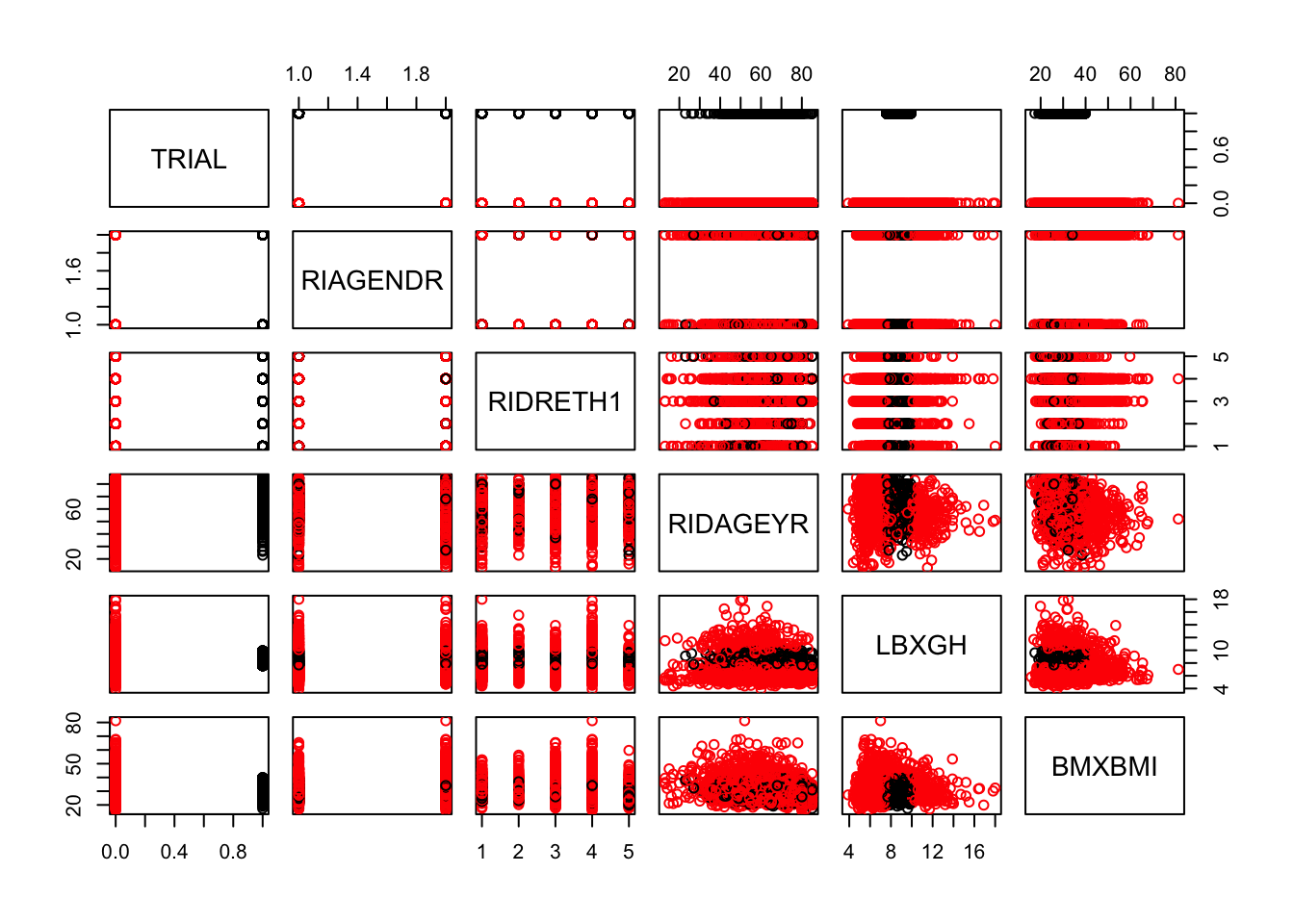``````# Build the logistic regression model
logi_fit = glm(TRIAL ~ RIAGENDR + RIDRETH1 + RIDAGEYR + LBXGH + BMXBMI, data = T2D, family = binomial)
summary(logi_fit) ``````
``````##
## Call:
## glm(formula = TRIAL ~ RIAGENDR + RIDRETH1 + RIDAGEYR + LBXGH +
##     BMXBMI, family = binomial, data = T2D)
##
## Deviance Residuals:
##     Min       1Q   Median       3Q      Max
## -2.6206  -0.6138  -0.4921  -0.3368   2.0689
##
## Coefficients:
##                             Estimate Std. Error z value Pr(>|z|)
## (Intercept)                -4.071891   0.529173  -7.695 1.42e-14 ***
## RIAGENDRFemale             -0.099573   0.105338  -0.945  0.34452
## RIDRETH1Other Hispanic     -0.195917   0.207811  -0.943  0.34580
## RIDRETH1Non-Hispanic White -0.168980   0.143165  -1.180  0.23787
## RIDRETH1Non-Hispanic Black -0.285172   0.150798  -1.891  0.05861 .
## RIDRETH1Other Race          0.040145   0.213599   0.188  0.85092
## RIDAGEYR                    0.013193   0.004540   2.906  0.00366 **
## LBXGH                       0.461420   0.030024  15.368  < 2e-16 ***
## BMXBMI                     -0.046595   0.008618  -5.407 6.42e-08 ***
## ---
## Signif. codes:  0 '***' 0.001 '**' 0.01 '*' 0.05 '.' 0.1 ' ' 1
##
## (Dispersion parameter for binomial family taken to be 1)
##
##     Null deviance: 2713.7  on 2694  degrees of freedom
## Residual deviance: 2366.0  on 2686  degrees of freedom
## AIC: 2384
##
## Number of Fisher Scoring iterations: 5``````
``````# RIDAGEYR, BMI and Glycohemoglobin values show significant p-values. Thus, we need to remove gender and ethnicity and update the model.

logi_fit2 = glm(TRIAL ~ RIDAGEYR + LBXGH + BMXBMI, data = T2D, family = binomial)
summary(logi_fit2) ``````
``````##
## Call:
## glm(formula = TRIAL ~ RIDAGEYR + LBXGH + BMXBMI, family = binomial,
##     data = T2D)
##
## Deviance Residuals:
##     Min       1Q   Median       3Q      Max
## -2.6542  -0.6091  -0.4971  -0.3405   2.0400
##
## Coefficients:
##              Estimate Std. Error z value Pr(>|z|)
## (Intercept) -4.090327   0.516348  -7.922 2.34e-15 ***
## RIDAGEYR     0.012122   0.004429   2.737   0.0062 **
## LBXGH        0.462886   0.029639  15.618  < 2e-16 ***
## BMXBMI      -0.050434   0.008365  -6.029 1.65e-09 ***
## ---
## Signif. codes:  0 '***' 0.001 '**' 0.01 '*' 0.05 '.' 0.1 ' ' 1
##
## (Dispersion parameter for binomial family taken to be 1)
##
##     Null deviance: 2713.7  on 2694  degrees of freedom
## Residual deviance: 2371.4  on 2691  degrees of freedom
## AIC: 2379.4
##
## Number of Fisher Scoring iterations: 5``````
``confint.default(logi_fit2) # 95% confidence intervals using standard errors``
``````##                    2.5 %      97.5 %
## (Intercept) -5.102350729 -3.07830258
## RIDAGEYR     0.003440768  0.02080271
## LBXGH        0.404795366  0.52097644
## BMXBMI      -0.066828015 -0.03403932``````
``exp(coef(logi_fit2)) # get the odds ratios``
``````## (Intercept)    RIDAGEYR       LBXGH      BMXBMI
##  0.01673377  1.01219550  1.58865207  0.95081700``````
``````# Check the mean RIDAGEYR, LBXGH, BMXBMI of trial participants and non-participants.
trial = T2D[which(T2D\$TRIAL==1),]
NonPart = T2D[which(T2D\$TRIAL==0),]
ave_age = cbind(mean(trial\$RIDAGEYR),mean(NonPart\$RIDAGEYR))
ave_LBXGH = cbind(mean(trial\$LBXGH),mean(NonPart\$LBXGH))
ave_BMI = cbind(mean(trial\$BMXBMI),mean(NonPart\$BMXBMI))
data = matrix(cbind(ave_age, ave_LBXGH,ave_BMI), ncol = 2, byrow = TRUE)
colnames(data) <- c("Trial Participants","Non-Participants")
rownames(data) <- c("Age","Hemoglobin A1C","BMI")
data = as.table(data)
data``````
``````##                Trial Participants Non-Participants
## Age                     63.291743        62.609302
## Hemoglobin A1C           8.494128         7.005674
## BMI                     30.084367        32.589112``````
``````# Trial participants have higher age than non-participants.
# Trial participants have higher Hemoglobin A1C values than non-participants.
# Trial participants have lower BMI than non-participants. ``````
• Linear Regression (Outcome is numerical)

Linear regression is a linear approach to modeling the relationship between a response and one or more predictor variables. The case of one predictor is called simple linear regression. For more than one predictors, the process is called multiple linear regression.

For example, we want to predict the Hemoglobin A1C value when age and BMI are known between the cilinical trial participants and non-participants.

``````# Building an initial linear regression model
lin_fit1 = lm(LBXGH ~ RIDAGEYR + BMXBMI + GROUP, data = T2D)
# Variable BMI is not significant. We need to remove it and update the model
summary(lin_fit1) ``````
``````##
## Call:
## lm(formula = LBXGH ~ RIDAGEYR + BMXBMI + GROUP, data = T2D)
##
## Residuals:
##     Min      1Q  Median      3Q     Max
## -3.5049 -0.8717 -0.3378  0.2809 10.7754
##
## Coefficients:
##              Estimate Std. Error t value Pr(>|t|)
## (Intercept)  8.302793   0.237482  34.962  < 2e-16 ***
## RIDAGEYR    -0.018766   0.002476  -7.578 4.78e-14 ***
## BMXBMI      -0.003750   0.004407  -0.851    0.395
## GROUPTrial   1.491867   0.078207  19.076  < 2e-16 ***
## ---
## Signif. codes:  0 '***' 0.001 '**' 0.01 '*' 0.05 '.' 0.1 ' ' 1
##
## Residual standard error: 1.615 on 2691 degrees of freedom
## Multiple R-squared:  0.1371, Adjusted R-squared:  0.1361
## F-statistic: 142.5 on 3 and 2691 DF,  p-value: < 2.2e-16``````
``````# Check the collinearity of predictors to make sure there are no two variales are highly correlated
# install.packages("olsrr")
library(olsrr)``````
``````##
## Attaching package: 'olsrr'``````
``````## The following object is masked from 'package:datasets':
##
##     rivers``````
``ols_vif_tol(lin_fit1) # There is no collinearity issue``
``````## # A tibble: 3 x 3
##   Variables  Tolerance   VIF
##   <chr>          <dbl> <dbl>
## 1 RIDAGEYR       0.944  1.06
## 2 BMXBMI         0.926  1.08
## 3 GROUPTrial     0.981  1.02``````
``````# A VIF of 1 means that there is no correlation among the kth predictor and the remaining predictor variables.
# The general rule of thumb is that VIFs exceeding 4 warrant further investigation, while VIFs exceeding 10 are signs of serious multicollinearity requiring correction.

# Remove BMI in lin_fit1 and refine the model as lin_fit2
lin_fit2 = update(lin_fit1, ~ RIDAGEYR + GROUP)
summary(lin_fit2)``````
``````##
## Call:
## lm(formula = LBXGH ~ RIDAGEYR + GROUP, data = T2D)
##
## Residuals:
##     Min      1Q  Median      3Q     Max
## -3.4389 -0.8725 -0.3351  0.2736 10.7822
##
## Coefficients:
##              Estimate Std. Error t value Pr(>|t|)
## (Intercept)  8.149411   0.154612  52.709  < 2e-16 ***
## RIDAGEYR    -0.018268   0.002406  -7.593 4.29e-14 ***
## GROUPTrial   1.500921   0.077476  19.373  < 2e-16 ***
## ---
## Signif. codes:  0 '***' 0.001 '**' 0.01 '*' 0.05 '.' 0.1 ' ' 1
##
## Residual standard error: 1.615 on 2692 degrees of freedom
## Multiple R-squared:  0.1369, Adjusted R-squared:  0.1362
## F-statistic: 213.5 on 2 and 2692 DF,  p-value: < 2.2e-16``````
``````# Use anova to compare the models with or without the variable BMI
anova(lin_fit1,lin_fit2)``````
``````## Analysis of Variance Table
##
## Model 1: LBXGH ~ RIDAGEYR + BMXBMI + GROUP
## Model 2: LBXGH ~ RIDAGEYR + GROUP
##   Res.Df    RSS Df Sum of Sq      F Pr(>F)
## 1   2691 7020.6
## 2   2692 7022.5 -1   -1.8891 0.7241 0.3949``````
``AIC(lin_fit1,lin_fit2)``
``````##          df      AIC
## lin_fit1  5 10238.42
## lin_fit2  4 10237.15``````
``````# The two models do not have much difference as p-value is greater than 0.05. Thus, we use the simpler model "lin_fit2".

## Implications ##

# GROUPTrial has a positive estimated coefficient = 1.5, with p-value less than 0.05.  It means that the Type 2 diabetes clinical trial are more likely to enroll patients with higher Hemoglobin A1C values.
# However, age has a negative estimated coefficient = -0.02, with p-value less than 0.05.  It means that patients who are younger are more likely to have higher Hemoglobin A1C values.``````

## 6. Survival Analysis for Outcomes or Mortality Comparisions

For survival analysis, the survival and survminer packages in R will be used. The ovarian dataset (Edmunson J.H. et al., 1979) that comes with the survival package will be used for the following analysis.

The ovarian dataset comprises a cohort of ovarian cancer patients and respective clinical information, including the time patients were tracked until they either died or were lost to follow-up (futime), whether patients were censored or not (fustat), patient age (age), treatment group assignment (rx), presence of residual disease (resid.ds) and ECOG performance status (ecog.ps).

• Kaplan-Meier estimator

The Kaplan-Meier estimator, independently described by Edward Kaplan and Paul Meier. It is a non-parametric statistic used to estimate the survival function from lifetime data. In medical research, it is often used to measure the fraction of patients living for a certain amount of time after treatment.

An important advantage of the Kaplan–Meier curve is that the method can take into account some types of censored data, particularly right-censoring, which occurs if a patient withdraws from a study, is lost to follow-up, or is alive without event occurrence at last follow-up.

In order to generate a Kaplan–Meier estimator, at least two pieces of data are required for each patient (or each subject): the status at last observation (event occurrence or right-censored) and the time to event (or time to censoring). If the survival functions between two or more groups are to be compared, then a third piece of data is required: the group assignment of each subject.

``````# install.packages("survival")
# install.packages("survminer")
library(survival)
library(survminer)``````
``## Loading required package: ggplot2``
``````##
## Attaching package: 'ggplot2'``````
``````## The following objects are masked from 'package:psych':
##
##     %+%, alpha``````
``## Loading required package: ggpubr``
``## Loading required package: magrittr``
``````# Import the ovarian cancer dataset
data(ovarian)
``````##   futime fustat     age resid.ds rx ecog.ps
## 1     59      1 72.3315        2  1       1
## 2    115      1 74.4932        2  1       1
## 3    156      1 66.4658        2  1       2
## 4    421      0 53.3644        2  2       1
## 5    431      1 50.3397        2  1       1
## 6    448      0 56.4301        1  1       2``````
``````# Dichotomize continuous variable "age" to binary values
hist(ovarian\$age)
# According to the histogram, devide patients into two groups who are below 50 or not
ovarian <- ovarian %>% mutate(age_group = ifelse(age >=50, "old", "young"))
ovarian\$age_group <- factor(ovarian\$age_group)
``````##   futime fustat     age resid.ds rx ecog.ps age_group
## 1     59      1 72.3315        2  1       1       old
## 2    115      1 74.4932        2  1       1       old
## 3    156      1 66.4658        2  1       2       old
## 4    421      0 53.3644        2  2       1       old
## 5    431      1 50.3397        2  1       1       old
## 6    448      0 56.4301        1  1       2       old``````
``````# Change data values to labels
ovarian\$rx <- factor(ovarian\$rx, levels = c("1", "2"), labels = c("A", "B"))
ovarian\$resid.ds <- factor(ovarian\$resid.ds, levels = c("1", "2"), labels = c("no", "yes"))
ovarian\$ecog.ps <- factor(ovarian\$ecog.ps, levels = c("1", "2"), labels = c("good", "bad"))
``````##   futime fustat     age resid.ds rx ecog.ps age_group
## 1     59      1 72.3315      yes  A    good       old
## 2    115      1 74.4932      yes  A    good       old
## 3    156      1 66.4658      yes  A     bad       old
## 4    421      0 53.3644      yes  B    good       old
## 5    431      1 50.3397      yes  A    good       old
## 6    448      0 56.4301       no  A     bad       old``````
``````# Fit survival data using the Kaplan-Meier method
surv_object <- Surv(time = ovarian\$futime, event = ovarian\$fustat)
surv_object ``````
``````##     59   115   156   421+  431   448+  464   475   477+  563   638   744+
##   769+  770+  803+  855+ 1040+ 1106+ 1129+ 1206+ 1227+  268   329   353
##   365   377+``````
``````# Examine prdictive value of the treatment groups
fit1 <- survfit(surv_object ~ rx, data = ovarian)
summary(fit1)``````
``````## Call: survfit(formula = surv_object ~ rx, data = ovarian)
##
##                 rx=A
##  time n.risk n.event survival std.err lower 95% CI upper 95% CI
##    59     13       1    0.923  0.0739        0.789        1.000
##   115     12       1    0.846  0.1001        0.671        1.000
##   156     11       1    0.769  0.1169        0.571        1.000
##   268     10       1    0.692  0.1280        0.482        0.995
##   329      9       1    0.615  0.1349        0.400        0.946
##   431      8       1    0.538  0.1383        0.326        0.891
##   638      5       1    0.431  0.1467        0.221        0.840
##
##                 rx=B
##  time n.risk n.event survival std.err lower 95% CI upper 95% CI
##   353     13       1    0.923  0.0739        0.789        1.000
##   365     12       1    0.846  0.1001        0.671        1.000
##   464      9       1    0.752  0.1256        0.542        1.000
##   475      8       1    0.658  0.1407        0.433        1.000
##   563      7       1    0.564  0.1488        0.336        0.946``````
``````# Plot the curves
ggsurvplot(fit1, data = ovarian)``````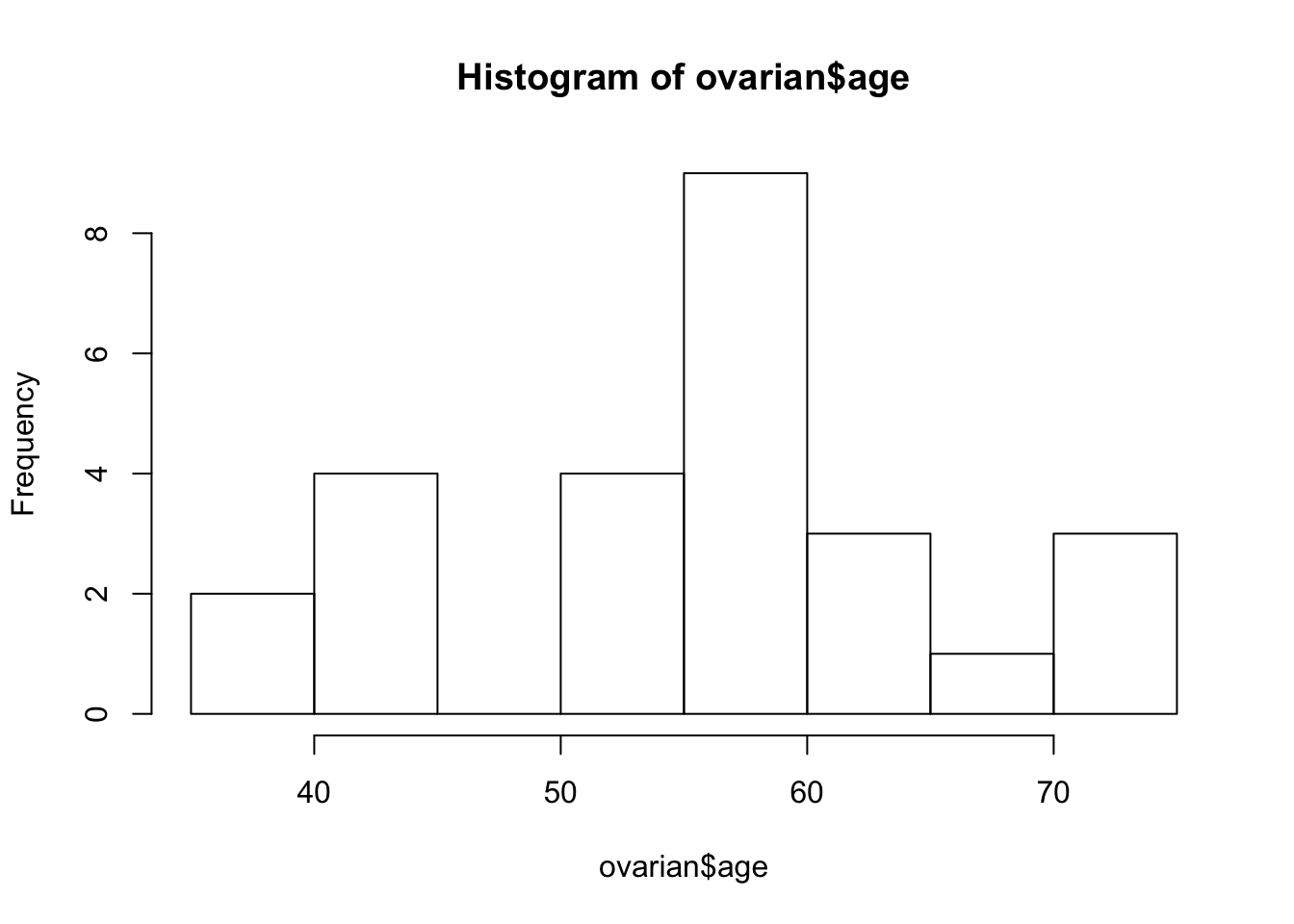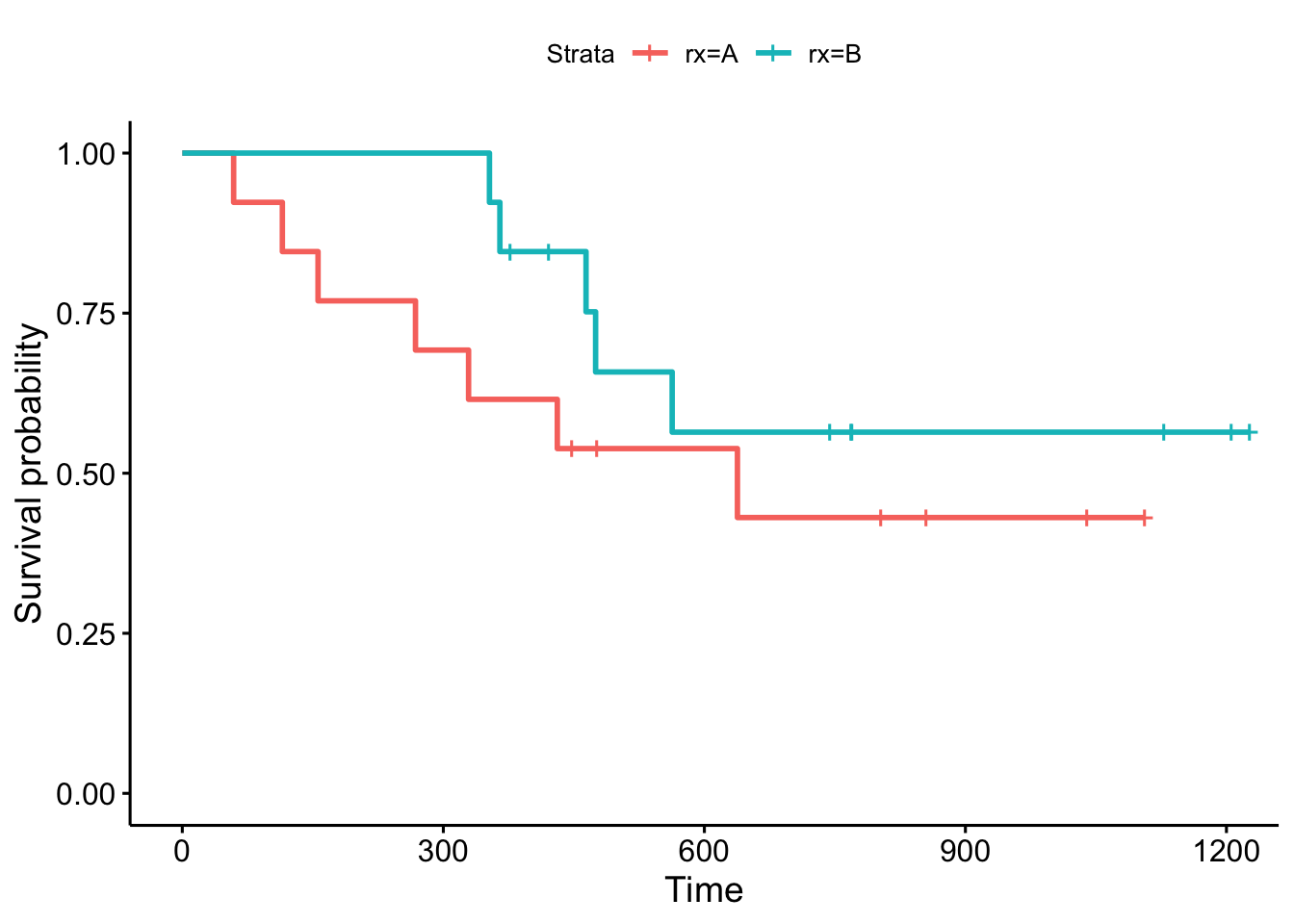``````# Examine prdictive value of residual disease status
fit2 <- survfit(surv_object ~ resid.ds, data = ovarian)
ggsurvplot(fit2, data = ovarian)``````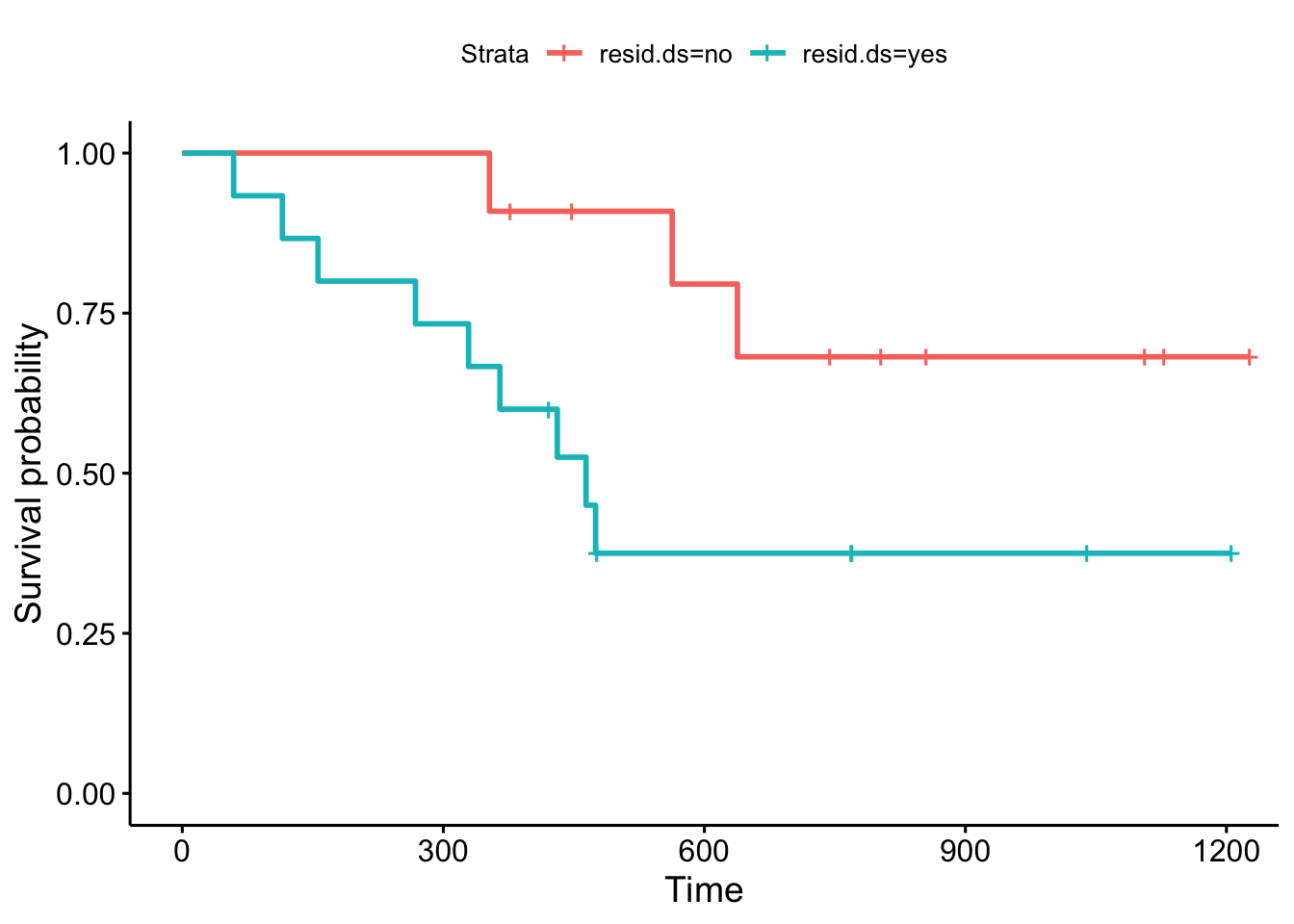``````# Examine prdictive value of the age groups
fit3 <- survfit(surv_object ~ age_group, data = ovarian)
ggsurvplot(fit3, data = ovarian)``````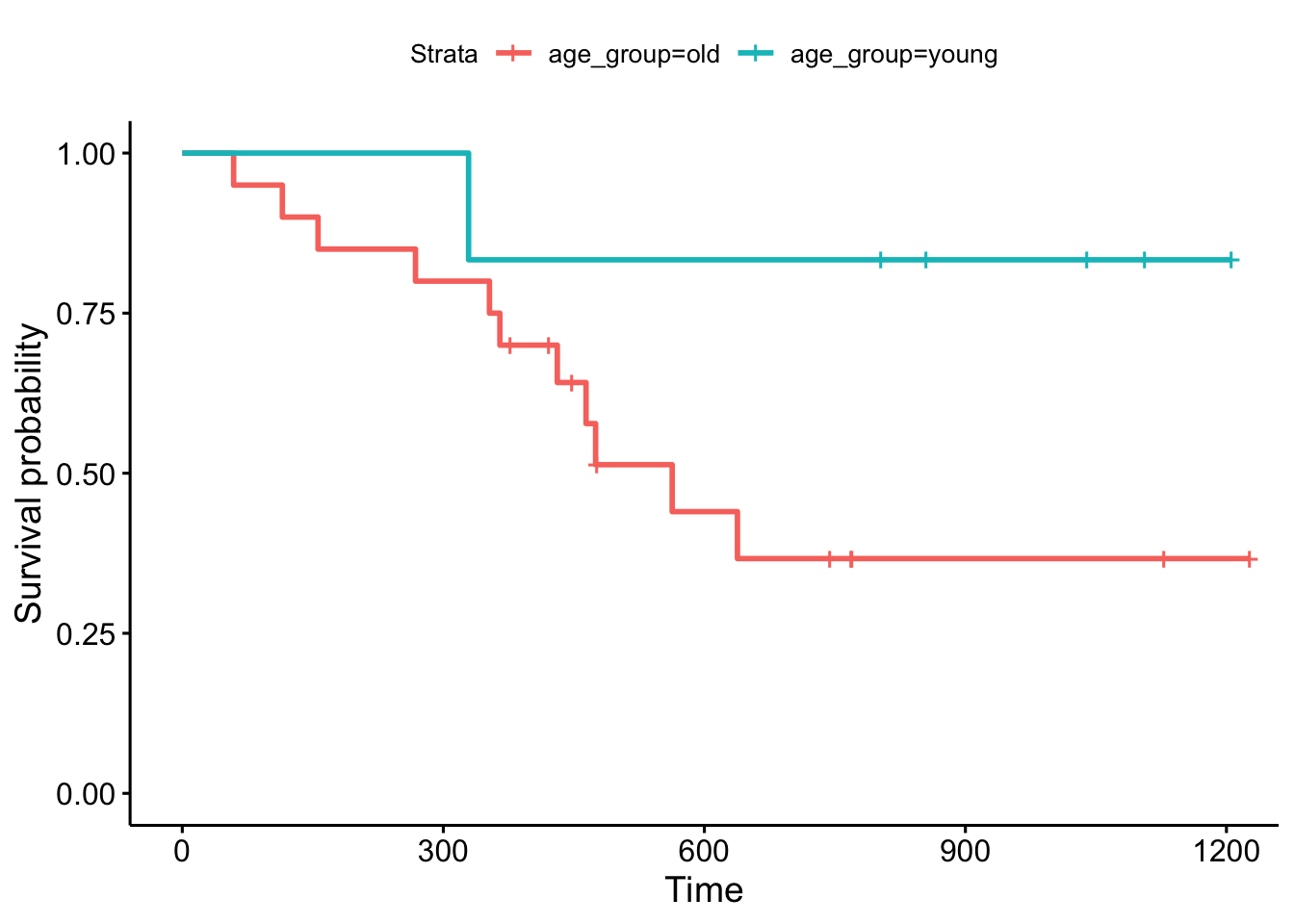• Log-rank test

The log-rank test can be used to compare survival curves of two groups. It is a statistical hypothesis test that tests the null hypothesis that survival curves of two populations do not differ.

``````# The log-ran test is simple when using the ggsurvplot() function
# The pval = TRUE argument conducts and plots the p-value of a log rank test in the Kaplan-Meier plot as well

# Compare the survial probabilities between the two treatment groups
ggsurvplot(fit1, data = ovarian, pval = TRUE)``````